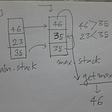# The Knapsack Problem (& a short introduction to Dynamic Programming)

Difficulty Level: Medium

# The Problem

The Knapsack Problem is an interesting problem of combinatorial optimization — given the weights and values of n items, determine the optimal combination of items within the given weight limit to maximize the total value in the knapsack.

The input to the problem is usually given by two integer arrays: value[0…n-1] and weight[0…n-1], as well as a weight limit W. In the problem above, the two arrays given are int[] value = {4, 2, 2, 1, 10} and int[] weight = {12, 2, 1, 1, 4}, and the given weight limit is 15kg.

# The Naive Method

The Knapsack Problem can be solved using a trivial, brute-force approach. Since there are n items given in the problem, there are a total of 2^n combinations of the items. To find the optimal combination that is within the given weight limit, simply search through all 2^n subsets and find the subset that maximizes the total value. However, this method can be extremely costly, and only works well for a small n number of items. Instead, we will use Dynamic Programming to derive a more efficient algorithm for the problem.

# Dynamic Programming

According to Wikipedia,

Dynamic Programming refers to simplifying a complicated problem by breaking it down into simpler sub-problems in a recursive manner.

It requires the problem to have an optimal substructure (i.e. if an optimal solution can be constructed from the optimal solutions of its subproblems), as well as overlapping subproblems. It is usually applied on problems that have a polynomial number of subproblems when expressed recursively, with a large number of overlaps among the subproblems causing it to run in exponential time.

In Dynamic Programming, the recursive solution is computed iteratively in a bottom-up fashion, avoiding wastage of computation and achieving a more efficient implementation.

# The Solution

Step One

Let m[i, j] be the maximum value that can be obtained using a subset of items in {1, 2, … i} and a total weight no more than j.

Let’s take a look at the two possible cases when adding the nth item (the last item) to the knapsack:

Case 1: The nth item is added to the knapsack

In this case, the maximum value m[i, j] can be given by m[i-1, j-wᵢ] + vᵢ, where wᵢ is the weight and vᵢ is the value of the nth item.

Case 2: The nth item is not added to the knapsack

In this case, the maximum value m[i, j] will be given by m[i-1, j].

Step Two

We can now derive an expression for m[i, j]:

If i = 0 or j = 0, then m[i, j] = 0.

If wᵢ ≤ j, then m[i, j] = max{(m[i-1, j-wᵢ] + vᵢ, m[i-1, j])}.

Otherwise, m[i, j] = m[i-1, j].

Step Three

Next, we construct a two-dimensional array (i.e. a table) with n + 1 rows and w + 1 columns. Each table value represents m[i, j], where i refers to the row index and j the column index. We initialize all values in the first row (i.e. index 0) and first column with the value 0.

Using the expression derived in step two, we can fill in the remaining table values starting from the first row, until we get the following table:

And voila, we are done! The maximum value that can be obtained with a given weight limit of 15kg is 15 (row n, column W).

Time Complexity

Since each table entry takes O(1) time, and there are a total of n x W table entries, the entire algorithm runs in O(nW) time — a huge improvement from O(2^n) using the naive method!

Converting to code

Note: The following is only pseudocode, challenge yourself to implement the working code on your own!

## More from Tan Yan Lyn

Love podcasts or audiobooks? Learn on the go with our new app.

## CSS | The Cascade: Importance## How To Change The Default Flutter App Icon For iOS And Android Adaptive Icons## Introduction to Functors in Typescript## Neo4j and Gephi Tool for Graphical Analysis of data## A Trip to the C Library. Creating Static Libraries## The A.I. Protocol## Sertizens in Action: Introducing our Senior Data Engineer — Jet Schuett## Queue | The Data Structure which Schedules the Disk## The Blind 75 Leetcode Series: Valid Parentheses## Design an algorithm that can return the Maximum item of a stack in O(1) running time complexity.